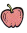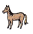# McGraw Hill Math Grade 1 Chapter 3 Lesson 6 Answer Key Subtraction Word Problems

All the solutions provided in McGraw Hill Math Grade 1 Answer Key PDF Chapter 3 Lesson 6 Subtraction Word Problems as per the latest syllabus guidelines.

## McGraw-Hill Math Grade 1 Answer Key Chapter 3 Lesson 6 Subtraction Word Problems

Solve

Subtract to solve the problems. Write the difference.

Question 1.
Sue has 8in a bag. Sue gives 3away. How manydoes Sue have now?
8 – 3 = 5 apples
Given that
Sue has 8in a bag. Sue gives 3away.
8 – 3 = 5 apples
Therefore Sue have 5 apples now.

Question 2.
There are 17in the field. 15go into the barn. How manyare in the field now?
17 – 15 = _____ horses
There are 17in the field. 15go into the barn.
17 – 15 = 2There are 2in the field now.